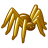# Why doesn't the annotation and the map match?

294
0
08-09-2017 12:32 PMNew Contributor

Why  doesn't  the annotation  and the map  match?

There are two arcgis map services.

The map service is http://mape.shanghai-map.net/ArcGIS/rest/services/SHMAP_D/MapServer.

The annotation   service   is   http://mape.shanghai-map.net/ArcGIS/rest/services/SHMAP_DZJ/MapServer.

The webgis which I use is openlayer3.

new ol.layer.Tile({
source: new ol.source.TileArcGISRest({
url: "http://mape.shanghai-map.net/ArcGIS/rest/services/SHMAP_D/MapServer"
})
}),
new ol.layer.Tile({
source: new ol.source.TileArcGISRest({
url: "http://mape.shanghai-map.net/ArcGIS/rest/services/SHMAP_DZJ/MapServer"
})
})

But  the  annotation   doesn't  match   the  map.

1、Does the annotation  has  to  import with  special method  in openlayer3?   Why  doesn't  the annotation  and the map  match?

I tried use  map tile of the two service, But I can't exactly calculate the Y value and I don't know the {z}/{y}/{x}?

I  tried use a function of openlayer3 to  calculate the z\ y\x value, but I can't  calculate the Y value  very accurate.

The code as below:

var source = new ol.source.XYZ({
wrapX: false,
// tileSize: 512,
tilePixelRatio: 2,
tileGrid: ol.tilegrid.createXYZ({}),
tileUrlFunction: function (tileCoord) {

const u = 'http://mape.shanghai-map.net/ArcGIS/rest/services/SHMAP_D/MapServer';
let z = tileCoord;
let x = tileCoord;
let y = -tileCoord - 1;

// let z = 'L' + (this.zeroPad(tileCoord, 2, 10));
// let x = 'C' + this.zeroPad(tileCoord, 8, 16);
// let y = 'R' + this.zeroPad(-tileCoord - 1, 8, 16);
return u + '/tile/' + (z - 10) + '/' + (y - Math.pow(2, z - 2) + Math.pow(2, z - 😎 + 1) + '/' + x;

}

2、Why should I have to  calculate the z\ y\x value?

How can I calculate the y-value right?

Tags (1)
0 Replies